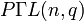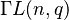# Projective semilinear group

## Definition

Let$K$ be a field and$n$ be a natural number.

The projective semilinear group or projective general semilinear group$P\Gamma L(n,K)$ is defined as the quotient group of the general semilinear group$\Gamma L(n,K)$ by the subgroup corresponding to scalar linear transformations, i.e., the subgroup corresponding to the center of$GL(n,K)$. (Note that the subgroup by which we are quotienting is not in the center of$\Gamma L(n,K)$ in general).

It can also be defined as an external semidirect product of the projective general linear group by a Galois group:$P\Gamma L(n,K) = PGL(n,K) \rtimes \operatorname{Gal}(K/k)$

where$k$ is the prime subfield of$K$.

For a prime power$q$, we denote by$P\Gamma L(n,q)$ the group$P\Gamma L(n,\mathbb{F}_q)$ where$\mathbb{F}_q$ is the (unique up to isomorphism) field of size$q$.

## Arithmetic functions

We consider here a field$K = \mathbb{F}_q$ of size$q = p^r$ where$p$ is the field characteristic, so$r$ is a natural number.

The prime subfield is$k = \mathbb{F}_p$, and the extension$K/k$ has degree$r$. The Galois group of the extension thus has size$r$. Note that the Galois group of the extension is always a cyclic group of order$r$ and is generated by the Frobenius automorphism$x \mapsto x^p$.

We are interested in the group$P\Gamma L(n,q)$.

Function Value Similar groups Explanation
order$rq^{\binom{n}{2}}\prod_{i=2}^n (q^i - 1)$ order of semidirect product is product of orders: we multiply the order$r$ of the Galois group with the order of the projective general linear group.

## Particular cases

We consider a field of size$q = p^r$ where$p$ is the underlying prime and field characteristic, and therefore$r$ is the degree of the extension over the prime subfield and also the order of the Galois group.

Note that in the case$r = 1$, the projective general semilinear group coincides with the projective general linear group.

In the case$n = 1$, the projective semilinear group is just the Galois group, which is cyclic of order$r$.

Finally, if$r = n = 1$, we get a trivial group.$q$ (field size)$p$ (underlying prime, field characteristic)$r$ (degree of extension over prime subfield)$n$$\Gamma L(n,q)$ order of$\Gamma L(n,q)$
2 2 1 1 trivial group 1
3 3 1 1 trivial group 1
4 2 2 1 cyclic group:Z2 1
5 5 1 1 trivial group 1
7 7 1 1 trivial group 1
8 2 3 1 cyclic group:Z3 3
9 3 2 1 cyclic group:Z2 1
2 2 1 2 symmetric group:S3 6
3 3 1 2 symmetric group:S4 24
4 2 2 2 symmetric group:S5 120
5 5 1 2 symmetric group:S5 120
7 7 1 2 projective general linear group:PGL(2,7) 336
8 2 3 2 Ree group:Ree(3) 1512
9 3 2 2 automorphism group of alternating group:A6 1440